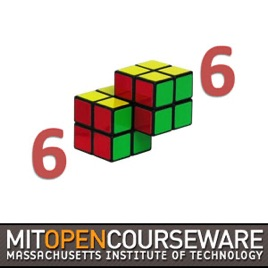47 episodios

Lecture videos from 6.006 Introduction to Algorithms, taught by Erik Demaine and Srini Devadas. The course is divided into eight units: introduction, sorting and trees, hashing, numerics, graphs, shortest paths, dynamic programming, and advanced topics.# Introduction to Algorithms (2011) MIT

• Tecnología

Lecture videos from 6.006 Introduction to Algorithms, taught by Erik Demaine and Srini Devadas. The course is divided into eight units: introduction, sorting and trees, hashing, numerics, graphs, shortest paths, dynamic programming, and advanced topics.